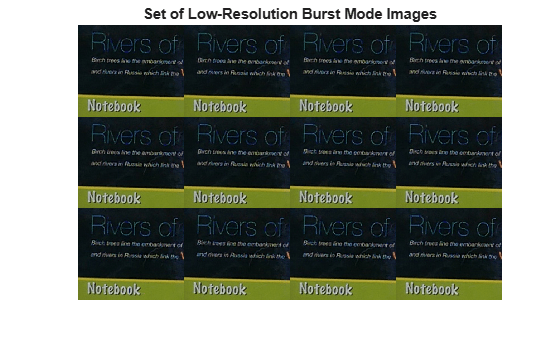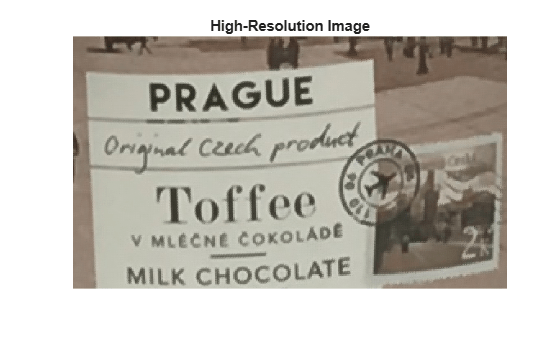# burstinterpolant

Create high-resolution image from set of low-resolution burst mode images

Since R2019a

## Syntax

``imHighRes = burstinterpolant(imds,tforms,scale)``
``imHighRes = burstinterpolant(images,tforms,scale)``

## Description

example

````imHighRes = burstinterpolant(imds,tforms,scale)` creates a high-resolution image, `imHighRes` from a set of low-resolution burst mode images stored as an `ImageDatastore` object, `imds`. The size of `imHighRes` is `scale` times the size of the input images. `tforms` specifies the geometric transformations required to align the low-resolution images.```

example

````imHighRes = burstinterpolant(images,tforms,scale)` creates a high-resolution image, `imHighRes` from a set of low-resolution burst mode images stored in cell array `images`.```

## Examples

collapse all

Specify the location of low-resolution burst mode images. The input images are 2-D RGB images with slight variations of rotation and translation.

`setDir = fullfile(toolboxdir("images"),"imdata","notebook");`

Use the `imageDatastore` function to read and store the low-resolution burst mode images.

`imds = imageDatastore(setDir,"FileExtensions",".png");`

Display the images as a montage.

```montage(imds) title("Set of Low-Resolution Burst Mode Images")```Calculate Geometric Transformations

Convert all the RGB images into lightness images by using the `rgb2lightness` function. The burst mode lightness images are stored as an image datastore object.

`imdsTransformed = transform(imds,@(x) rgb2lightness(x));`

Get the optimal configuration parameters required for registration of the burst mode lightness images by using the `imregconfig` function. Specify the image capture modality as "`monomodal"`.

`[optimizer,metric] = imregconfig("monomodal");`

Find the total number of images stored in the image datastore object by using the `numpartitions` function.

`numImages = numpartitions(imds);`

Create an array of 2-D affine transformation objects to store the rigid transformations of each low-resolution burst mode lightness image excluding the reference image. Set the number of rows in the transformation array as total number of images in the image datastore object minus one.

`tforms = repmat(affinetform2d,numImages-1,1);`

Read the first lightness image into the workspace and use it as the reference image for estimating the geometric transformations.

`imgRef = read(imdsTransformed);`

Use the `imregtform` function to estimate a rigid geometric transformation for each low-resolution burst mode lightness image with respect to the reference image.

```idx = 1; while hasdata(imdsTransformed) imgMoving = read(imdsTransformed); tforms(idx) = imregtform(imgRef,imgMoving,"rigid",optimizer,metric); idx = idx+1; end```

Create High-Resolution Image

Specify a scale factor for generating the high-resolution image.

`scale = 4;`

Create the high-resolution image from the set of low-resolution burst mode RGB images.

`imgHighRes = burstinterpolant(imds,tforms,scale);`

Display the high-resolution image.

```figure("WindowState","maximized") imshow(imgHighRes) title("High-Resolution Image")```Display the size of a low-resolution image.

```imgLowRes = read(imds); inputDim = size(imgLowRes)```
```inputDim = 1×3 161 186 3 ```

Display the size of the high-resolution image. Because the scale factor is 4, the spatial dimensions of the high-resolution image are 4 times the size of the low-resolution burst mode RGB images.

`outputDim = size(imgHighRes)`
```outputDim = 1×3 644 744 3 ```

Load a cell array containing the low-resolution burst mode images. The input images are 2-D RGB images with slight variations of rotation and translation.

`load("LRData")`

Display the images as a montage.

```montage(images,"Size",[2 4],"BackgroundColor",[1 1 1]) title("Set of Low-Resolution Burst Mode Images")```Calculate Geometric Transformation Parameters

Convert all the RGB images into lightness images by using the `rgb2lightness` function.

`imagesT = cellfun(@rgb2lightness,images,"UniformOutput",false);`

Get the optimal configuration parameters required for registration of the burst mode lightness images by using the `imregconfig` function. Specify the image capture modality as "`monomodal"`.

`[optimizer,metric] = imregconfig("monomodal");`

Find the total number of images stored in the cell array.

`numImages = length(images);`

Create an array of 2-D affine transformation objects to store the rigid transformations of each low-resolution burst mode lightness image excluding the reference image. Set the number of rows in the transformation array as total number of images in the image datastore object minus one.

`tforms = repmat(affinetform2d,numImages-1,1);`

Read the first lightness image into the workspace and use it as the reference image for estimating the geometric transformations.

`imgRef = imagesT{1};`

Use the `imregtform` function to estimate a rigid geometric transformation for each low-resolution burst mode lightness image with respect to the reference image.

```for i= 2:length(images) imgMoving = imagesT{i}; tforms(i-1) = imregtform(imgRef,imgMoving,"rigid",optimizer,metric); end```

Create High-Resolution Image

Specify the scale factor for generating the high-resolution image.

`scale = 3;`

Construct the high-resolution image from the set of low-resolution burst mode RGB images.

`imgHighRes = burstinterpolant(images,tforms,scale);`

Display the high-resolution image.

```imshow(imgHighRes) title("High-Resolution Image")```Display the size of a low-resolution image.

```imgLowRes = images{1}; inputDim = size(imgLowRes)```
```inputDim = 1×3 154 265 3 ```

Display the size of the high-resolution image. Because the scale factor is 3, the spatial dimensions of the high-resolution image are 3 times the size of the low-resolution image.

`ouputDim = size(imgHighRes)`
```ouputDim = 1×3 462 795 3 ```

## Input Arguments

collapse all

Input image datastore, specified as an `ImageDatastore` object. The input image datastore contains multiple low-resolution burst mode images used for creating the high-resolution image output.

• Images in the input image datastore must be 2-D grayscale images of size m-by-n or 2-D RGB images of size m-by-n-by-3.

• All images in the input image datastore must be of the same size and data type.

• The number of images in the input image datastore must be greater than or equal to 2.

• The images must be of data type `single`, `double`, `uint8`, or `uint16`.

Input images, specified as a k-by-1 cell array. k is the number of input images stored in the cell array. All of the input images must have same size.

Data Types: `single` | `double` | `uint8` | `uint16`

Geometric transformation, specified as a vector of geometric transformation object listed in the table. The vector has length k-1, where k is the number of images in `imds` or `images`. The `n`-th element of `tforms` indicates the geometric transformation used to register the `(n+1)`-th low-resolution image in `imds` or `images` to the first image.

Geometric Transformation ObjectDescription
`transltform2d`Translation transformation
`rigidtform3d`Rigid transformation: translation and rotation
`simtform2d`Similarity transformation: translation, rotation, and isotropic scaling
`affinetform2d`Affine transformation: translation, rotation, anisotropic scaling, reflection, and shearing

You can calculate the geometric transformation for each input image using the `imregtform` function. Specify the reference image as the first image in `imds` or `images`.

Note

You can also specify `tform` as a vector of `affine2d` objects. However, these objects are not recommended. For more information, see Compatibility Considerations.

Resize factor, specified as a numeric scalar greater than or equal to 1.

## Output Arguments

collapse all

High-resolution image, returned as a 2-D grayscale image or 2-D RGB image. `imHighRes` is of the same data type as the input images. The size of `imHighRes` is the value of `scale` times the size of the images in `imds` or `images`.

For example, let L be the value of `scale`, and m-by-n be the size of the low-resolution burst mode images. Then, the size of the high-resolution image is mL-by-nL.

## Algorithms

The `burstinterpolant` function uses the inverse distance weighting method to generate a high-resolution image from a set of low-resolution burst mode images . The use of geometric transformations `tforms` makes the pixel selection robust to rigid geometric transformations (rotations and translations only).

Note

• If the input images are 2-D RGB images, estimate `tforms` from the lightness component. You can use the `rgb2lightness` function to compute lightness values from the RGB color values.

 Shepard, Donald. “A Two-Dimensional Interpolation Function for Irregularly-Spaced Data”, In Proceedings of the 1968 23rd ACM National Conference, 517-524. New York, NY: ACM, 1968.